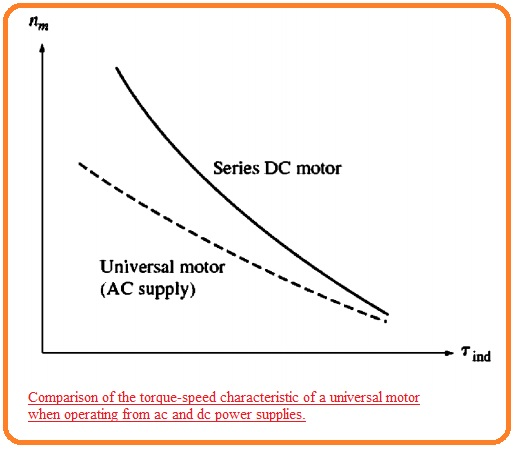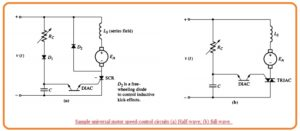Hi friends I hope you all are doing great. In this post, we will have a detailed look at Introduction to Universal Motor. The universal motor belongs to the special category of motors that created to operates through the DC or single-phase AC power supply. Their structure is such that they show the series-wound configuration and due to this configuration generated a high value of starting torque. Their operating speed is in the range of thirty-five hundred revolutions per minute.

As there is reactance losses are exist in this motor for ac supply so it operated at less speed than dc supply where these losses do not exist. In this post, we will have a detailed look at its working, operation, and some other related parameters. So let’s get started with Introduction to Universal Motor.

## Introduction to Universal Motor

• The most basic technique is to create a motor that can be operated from an ac supply having a dc configuration.
• The value of induced torque for dc motor can be found through it.

Tind=KIAØ

• If we altered the terminals of voltage provided to the shunt or dc series motor varied. then in results, the direction of field flux and direction of armatures current will also varied and the direction of torque produced will remain same as before.
• So it must be certain to get the single direction torque from the dc having pulses having ac as input.
• Similar to this design configuration can be seen here. That is exists for the series dc motor because the armature current and field current in this motor can be altered at the same point.
• In case of shunt dc motor, the large field inductance caused to delay the universal of IF and in results to decreases the average induced torque of the machine.
• For dc series dc motor to operate accurately at ac supply its fields poles and stator structure should have lamination. If there is not completed lamination exist their core losses will increase.
• In case of poles and stator laminations, this motor is known as a universal motor because it can operate from dc and ac supplies.
• When the motor is operating at ac supply the commutation will be poor in case of dc source.
• The additional sparking at brushes results in the transformer principle that generates induced voltage in the windings due to commutation.
• Due to these sparks, the operating life of the brush will decreases and results in radio frequency interference.### Torque-Speed characteristic of Universal Motor

• The certain torque-speed characteristic of the universal motor can be seen here.• It is not similar to the machine running through the dc supply due to 2 causes.
• The armature and field windings have a high value of reactance at fifty or sixty-hertz frequency. A certain portion of input voltage is lost about the reactances and so internal generated voltage EA is less for input voltage due to ac than due to dc.
• The peak voltage of ac network is √2 times its root mean square voltage therefore magnetic saturation can exist close to peak current in the motor.
• This saturation can significantly reduce the RMS flux of the motor for a given value of current causes to decrease the induced torque of the motor.
• Due to flux decrement speed of dc motor increases therefore this factor can be offset the speed reduce produced by the first point.

### Universal Motors Applications

• This motor provides the sharp decreases torque-speed characteristic of dc series motor due to this effect it is not preferred for constant speed applications.
• Therefore it is providing a high value of torque than any kind of single-phase motor.
• It preferred for less weight and high value of torque applications. Generally, this motor is used in vacuum, cleaners, drills kitchen appliances.

### Speed Control of Universal Motor

• The basic method to control the speed of the universal motor is to change the rms input voltage.
• The larger the value of rms input voltage the larger the speed of motor. The torque-speed characteristic of the universal motor can be seen here.• In practical the average voltage provided to this motor is changed through the usage of SCR circuits.
• In below figure these circuits of speed control are shown here.• The variable resistance can be seen here is the speed of adjustments of knows of motors.

That is all about the universal motor i tried to cover each and every parameter related to this device if you have any further query ask in the comments. Thanks for reading have a nice day.